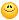Bitpacking Normal Vector Author Message
ChrisMember

 Post: #1 Bitpacking Normal Vector Hi, I'm using voxels, and each voxel has 4 bytes of data (32-bit). Previously I had my method working where a normal vector was packed into 3-bits, and the final bit stored some other data. But now I find that my paper can greatly be improved if I use 2 bits to encode the normal vector, and 2 bits for the other data. So I need to crudely encode a Vec normal; (magnitude of 1) as just 2-bytes, then be able to do the inverse - from 2 bytes, get a Vec normal again. This is my guess so far (using 5 bytes for x, 6 bytes for y, 5 bytes for z), but i'm not sure if it's correct - it's kinda hard to debug. Code: ```U16 nrmval =  (((U16)((in.normal.x+1)*16) << 11 ) & 0xFF) | (((U16)((in.normal.y+1)*16) << 6) & 0xFF) | ((U16)((in.normal.z+1)*16) & 0xFF); // pack normal Byte a = nrmval >> 8; Byte b = nrmval & 0xff;``` 1. I'm sure this is wrong, any ideas how to do it correctly? 2. I'm also not sure how to do the inverse. (This post was last modified: 03-03-2011 12:55 AM by Chris.)
02-27-2011 12:14 PM
ChrisMember

 Post: #2 RE: Bitpacking Lookup Table *Bumps this* I changed the original question quite a bit.
03-02-2011 06:18 PM
rndbitMember

 Post: #3 RE: Bitpacking Lookup Table maybe if you could explain it better.. i find hard time understanding exactly what you are trying to achieve. example in form of detailed expected input and output would help
03-02-2011 08:05 PM
ChrisMember

 Post: #4 RE: Bitpacking Lookup Table (03-02-2011 08:05 PM)rndbit Wrote:  maybe if you could explain it better.. i find hard time understanding exactly what you are trying to achieve. example in form of detailed expected input and output would help Okay: Input: Vec (96-bits) Output: Byte a; Byte b; (or a U16, it doesn't matter [16-bits]) The input is a normal vector, e.g. it has a length/magnitude of 1. This means that perhaps you can take advantage of it and use spherical coordinates: http://mynameismjp.wordpress.com/2009/06...ordinates/ http://aras-p.info/texts/CompactNormalStorage.html But in the above two links, they usually convert from Vec3 (96-bit) to Vec2 (64-bit), or from Half3 (48-bit), to Half2 (32-bit). 1. What I want to do is much more lossy & crude, encode from 96-bits to 16-bits, hopefully while taking advantage of the normal properties. 2. I'd like to also do the inverse; to decode from two bytes, to a Vec.
03-03-2011 12:41 AM
EsenthelPost: #5 RE: Bitpacking Normal Vector Code: ```Vec v; // v is normalized Byte x=Round(Lerp(0,255,LerpRS(-1,1,v.x))), // 8-bit prec      y=Round(Lerp(0,127,LerpRS(-1,1,v.y))); // 7-bit prec if(v.z<0)y|=128; // encode z sign in last y bit decode: v.x=Lerp(-1,1,LerpRS(0,255,x    )); v.y=Lerp(-1,1,LerpRS(0,127,y&127)); // length(v)==1 -> Sqrt(v.x*v.x + v.y*v.y + v.z*v.z)==1 -> v.x*v.x + v.y*v.y + v.z*v.z==1 -> v.z*v.z==1 - v.x*v.x - v.y*v.y -> v.z==Sqrt(1 - v.x*v.x - v.y*v.y) v.z=Sqrt(Sat(1 - v.x*v.x - v.y*v.y)); if(y&128)CHS(v.z); // if encoded z sign bit in y is on then change sign of v.z v.normalize();```
03-03-2011 04:10 AM
ChrisMember

 Post: #6 RE: Bitpacking Normal Vector Thank you so much Esenthel, this is so wonderfulIt works beautifully; can I ask how it works to know what to write about - how can you encode the z in just one sign bit? The quality seems very high.
03-03-2011 01:54 PM
EsenthelPost: #7 RE: Bitpacking Normal Vector only X Y are encoded, while for Z only it's sign is encoded. since you said that you need normal vector, then its length is 1, so having X Y you can calculate Z from following formula: length(v)==1 -> Sqrt(v.x*v.x + v.y*v.y + v.z*v.z)==1 -> v.x*v.x + v.y*v.y + v.z*v.z==1 -> v.z*v.z==1 - v.x*v.x - v.y*v.y -> v.z==Sqrt(1 - v.x*v.x - v.y*v.y) v.z=Sqrt(Sat(1 - v.x*v.x - v.y*v.y)); sqrt always gives positive value, but Z can be + or -, so we need to encode it's sign first (that's the last bit in 2nd byte for)
03-03-2011 02:20 PM
ChrisMember

 Post: #8 RE: Bitpacking Normal Vector Awesome! Such an elegant solution to the problem03-03-2011 02:44 PM
ChrisMember

 Post: #9 RE: Bitpacking Normal Vector Hi, I updated my question with my latest development of the problem: Here's what I have, a set of 3d vectors in a 3d flow.But they face different directions in the flow (backwards and forwards, left and right). I need to generate a lookup table of n random colours for each possible normal vector within some degree of accuracy (e.g. a sphere of some resolution where each vertex contains a colour), where opposite normal vectors (e.g. change sign) have the same colour. I then, idealistically (but not necessarily) need a function which finds the nearest normal vector & corresponding colour in this table, from a given normal vector. Input: normal vector Output: colour for corresponding closest normal vector -> where all opposite vectors in the "lookup sphere" have the same colour. Edit: Ah, nevermind - i've got this mostly working now. Thanks, Chris (This post was last modified: 04-23-2011 12:03 AM by Chris.)
04-22-2011 09:45 PM
 « Next Oldest | Next Newest »